##1. Line Involution through complete quadrilateral

There is a basic figure by which we define involutive homographies on lines. This is done by intersecting a line (e) with the six sides of a complete quadrilateral. The three pairs of points (X1,X2), (Y1,Y2) and (Z1,Z2) intercepted on (e) by corresponding pairs of opposite sides of the quadrilateral are in involution. This means that the involution F on e defined by two pairs (out of the three), (X1,X2), (Y1,Y2) say, interchanges also the points of the other pair (Z1,Z2).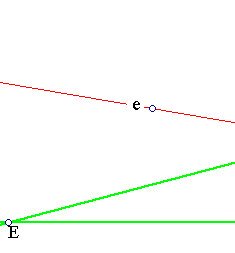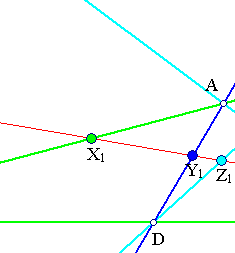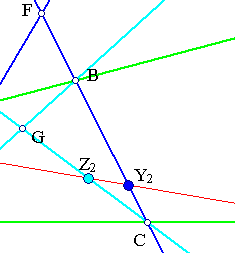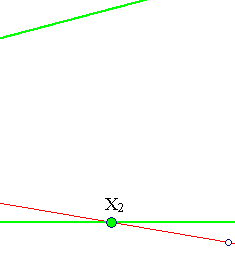To prove F(Z1) = Z2 it suffices to show the equality of cross ratios: (Y1,Y2,X1,Z1) and (Y2,Y1,X2,Z2). This follows from the preservation of cross ratios by F, by which
(Y1,Y2,X1,Z1) = (F(Y1),F(Y2),F(X1),F(Z1)) = (Y2,Y1,X2,F(Z1).
But the claimed equality can be read off from the figure.
(Y1,Y2,X1,Z1) = (Y1,F,A,D) (perspective from B)
(Y2,Y1,X2,Z2) = (F,Y1,D,A) (perspective from C).

The property can be extended to every conic passing through {A,B,C,D}. In fact, the set of two lines through G is a degenerate conic (passing through the four points) and its intersection points {Z1,Z2} with line (e), as we saw, are in involution with respect to F, defined by the two other pairs of points (X1,X2) and (Y1,Y2). See DesarguesInvolution.html for the details.
There is another aspect of the involution on (e) defined by the quadrilateral, connected with a circle bundle defined by the three circles with diameters X1X2, Y1Y2 and Z1Z2. This is discussed in InvolutionBasic2.html .

##2. Construction of the sixth point

An involution x'=F(x) on a line (e) is defined by giving two pairs of corresponding points, such as (Y1,Y2=F(Y1)) and (Z1,Z2=F(Z1)). Then the above figure can be used to construct the image X2=F(X1) of any fifth point X1 on line (e).
In fact, construct an arbitrary triangle ABD whose sides pass through {X1,Y1,Z1}. Then draw lines {AZ2,BY2} as in the figura above. These lines intersect at C and X2 results as intersection point of (e) with line CD.

##3. Cevians characterization through involutions

Given a triangle ABC one can characterize the coincidence at a point D of three lines {a',b',c'} emanating from its vertices using the previous property:
The three sides {a,b,c} of ABC and the three lines {a',b',c'} emanating from the vertices of ABC (as shown below) coincide in a point D precisely when lines {a,a',b,b',c,c'} intersect an arbitrary line (e) not passing through points {A,B,C,D} at points {X1,X2,Y1,Y2,Z1,Z2} which are pairwise in involution.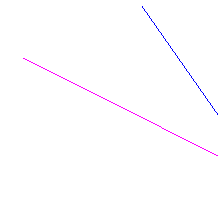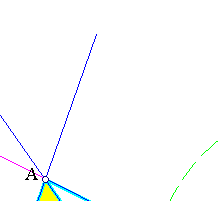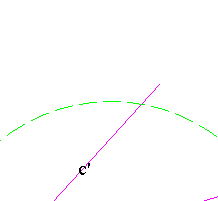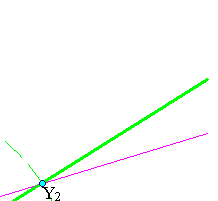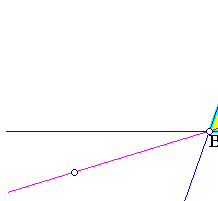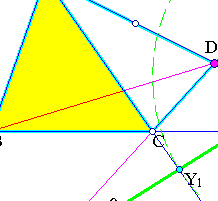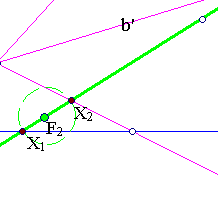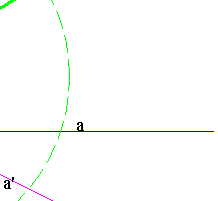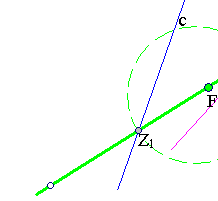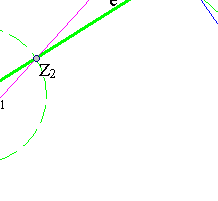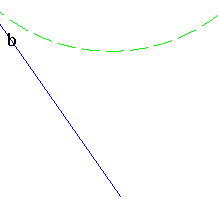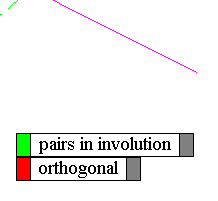The necessity of the condition follows directly from the previous property by considering the complete quadrilateral defined by the four points {A,B,C,D}.
The inverse (sufficiency) follows by the usual argument in such cases as those of Menelaus, Ceva, Desargues, in which use of the proof of necessity is made:
Assume the condition is satisfied and lines {a',b'} (only the two) intersect at D. Draw then through D line c''=AD and apply the necessity proof to {a',b',c''} and their intersection points {X2,Y2,W2} with line (e). It follows that {X1,X2,Y1,Y2,Z1,W2} are in involution. Since this involution is uniquely determined from the two first pairs {X1,X2,Y1,Y2} it coincides with the assumed involution of the pairs {X1,X2,Y1,Y2,Z1,Z2}. This implies W2=Z2 and the coincidence of c'' with c'.

### See Also

DesarguesInvolution.html
DesarguesInvolution2.html
DesarguesInvolution3.html
DesarguesInvolutionComplex.html
Involution.html
InvolutionBasic2.html
InvolutionBasic3.html

 Produced with EucliDraw© http://users.math.uoc.gr/~pamfilos/